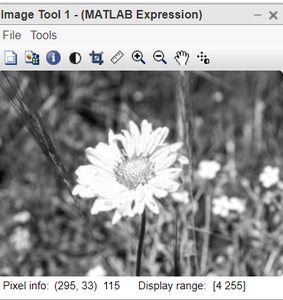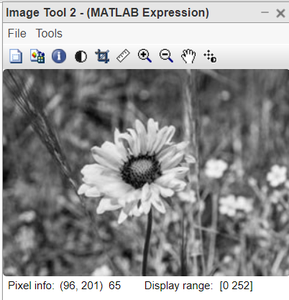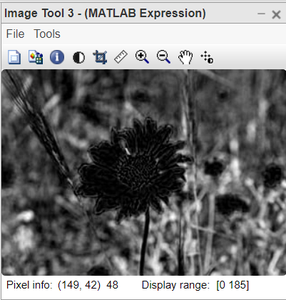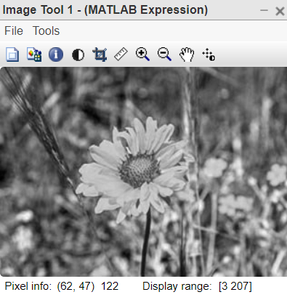# How to Convert Three Channels of Colored Image into Grayscale Image in MATLAB?

• Difficulty Level : Expert
• Last Updated : 16 Nov, 2021

In this article, we explore the RGB colour model conversion into grayscale.

Grayscale image: Grayscale is a range of gray shades from white to black, as used in a monochrome display or printout. Grayscale images are most commonly used in image processing because smaller data enables developers to do more complex operations in a shorter time.

Gray image contains 255 shades of black colour. 0 intensity represents black and 255 represents white shade. In between, there exist shades of dark and white. As we increase the number from 0 to 255, the dark colour fades and white adds up. Gray images are often called black and white images. Earlier cameras were capable of capturing only gray images.

RGB image: RGB is one of the colour models for representing coloured images. RGB stands for Red, Green and Blue. An RGB image contains 3 channels each for RGB colour. It is represented by three 2-D matrices. In the RGB model, the intensity values range from 0 to 255. Intensity 0 means dark Red, Green or Blue colour. As the intensity increases the colour fades and whiteness adds up. RGB intensity 0 represents black and 1 represents white colour.

```RGB value 0 (black) means Red=Green=Blue=0
RGB value 1 (white) means Red=Green=Blue=255```

There are a number of commonly used methods to convert an RGB image to a grayscale image such as

•  Average method
• Weighted method

Average method: The Average method takes the average value of R, G, and B as the grayscale value.

`Grayscale = (R + G + B ) / 3`

There may be some overflow error occurred if the sum of R, G, and B is greater than 255. To avoid the exception, R, G, and B should be calculated respectively.

`Grayscale = R / 3 + G / 3 + B / 3`

The average method is simple but doesn’t work as well as expected. The reason is that human eyeballs react differently to RGB. Eyes are most sensitive to green light, less sensitive to red light, and the least sensitive to blue light. Therefore, the three colours should have different weights in the distribution. That brings us to the weighted method.

Weighted method: The Weighted Method, also called the luminosity method, weighs red, green and blue according to their wavelengths. The improved formula is as follows:

` Grayscale = 0.299R + 0.587G + 0.114B`

### Convert RGB into Grayscale:

MatLab has an inbuilt function for this purpose which uses a weightage for RGB channels. But we can simply convert RGB into grayscale by taking the average of Red, Green and Blue channels.Function used :

• Display the image using imtool() function.
• Access particular colour channel: k(:, :, 1);

Example:

## Matlab

 `% Code in MatLab for separating Red,``% Green and Blue components.``% Read the colour image.``k=imread(``"yellow1.jpg"``);`` ` `% display original image.``imtool(k,[]);`` ` `% display Red channel.``imtool(k(:,:,1),[]);`` ` `% display Green channel.``imtool(k(:,:,2),[]);`` ` `% display Blue channel.``imtool(k(:,:,3),[]);`

Output:Figure 1:Original  Colour imageFigure 2: Output of Red ChannelFigure 3: Output of Green ChannelFigure 4: Output of Blue Channel

Code Explanation:

• imtool(k,[]); this line displays original image.
• imtool(k(:,:,1),[]); this line displays Red channel.
• imtool(k(:,:,2),[]);  this line displays Green channel..
• imtool(k(:,:,3),[]); this line displays Blue channel

## Matlab

 `% MATLAB code for convert RGB into Grayscale.``% read the image.``k=imread(``"yellow1.jpg"``);`` ` `%Convert into double format.``k=double(k);`` ` `% take the average of R, G and B channels.``k1=(k(:,:,1)+k(:,:,2)+k(:,:,3))/3;`` ` `% display after converting into uint8 format.``imtool(uint8(k1),[]);`

Output:Figure 5: Colour imageFigure 6: Grayscale image

Code explanation: